Mole Calc by David Defoort, the desk calculator you need for all chemicals !
Also available in French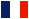[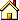Other software by David Defoort ]

 Latest News
New features: allows spaces into the formula, disable the Calculate button when the formula field is empty, empty the result field when the formula is modified.
February 07, 2000Release of MoleCalc 1.0.

 Mole Calc calculates the molecular weight of all your chemicals
Mole Calc is a user friendly Windows (95/98/NT) tool which is very similar to a classical calculator, but recognizes the atomic compounds from the Mendeleiev table, and knows their weight.
Some of the main features:
• Very easy to use interface,
• Accurate results,
• Accepts long and complicated chemical formula,
• Knows the atomic weight of unusual compound,
• Easy installation and uninstallation,
• Very useful for chemists, researcher, engineers, students...

• How does Mole Calc work?

• The interface is very simple to use. In fact, it can not be simpler! Let's take an example.

To calculate the molecular mass of the ethanol whose formula is CH3CH2OH, simply enter the formula in the Formula field and click on the Calculate button (you may also just press the Enter key):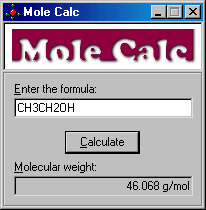• Is Mole Calc case sensitive?

• Yes, it has to be as chemical formulas are: Co and CO are totally different; Co, stands for Cobalt, and CO stands for Carbon and Oxygen: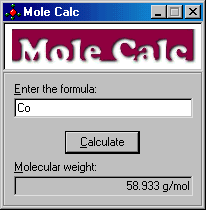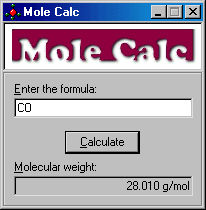• May I use parenthesis to write the formula?

• Sure, see the formula of heptanol on the next window!•Download Mole Calc 1.0.2 here (234 kb)